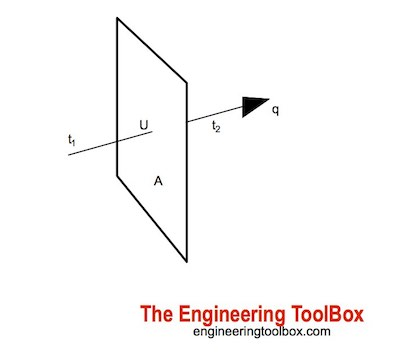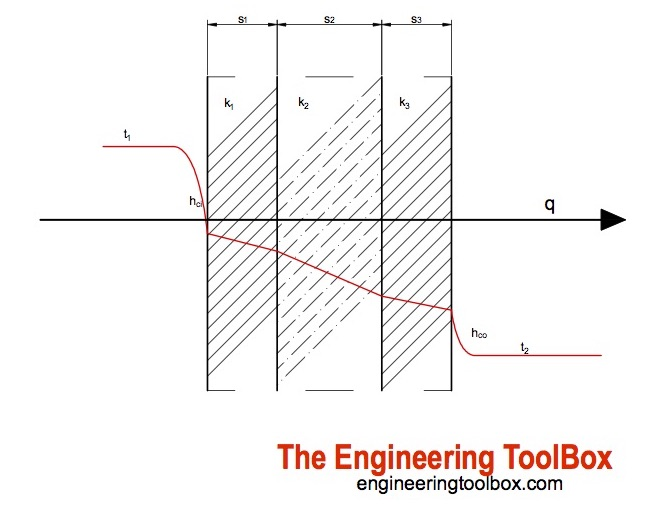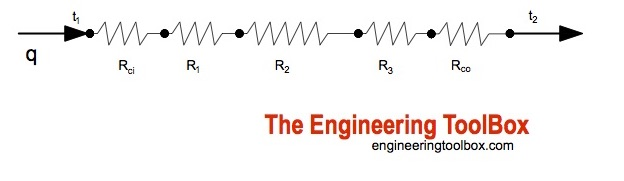Engineering ToolBox - Resources, Tools and Basic Information for Engineering and Design of Technical Applications!

# Overall Heat Transfer Coefficients

## Walls or heat exchangers - calculate overall heat transfer coefficients.Heat transfer through a surface like a wall can be calculated as

q = U A dT                        (1)

where

q = heat transfer (W (J/s), Btu/h)

U = overall heat transfer coefficient (W/(m2K), Btu/(ft2 h oF))

A = wall area (m2, ft2)

dT = (t1 - t2)

= temperature difference over wall (oC, oF)

The overall heat transfer coefficient for a multi-layered wall, pipe or heat exchanger - with fluid flow on each side of the wall - can be calculated as

1 / U A = 1 / hci Ai + Σ (sn / kn An) + 1 / hco Ao                                      (2)

where

U = the overall heat transfer coefficient (W/(m2 K), Btu/(ft2 h oF))

kn = thermal conductivity of material in layer n  (W/(m K), Btu/(hr ft °F))

hc i,o = inside or outside wall individual fluid convection heat transfer coefficient (W/(m2 K), Btu/(ft2 h oF))

sn = thickness of layer n (m, ft)

A plane wall with equal area in all layers - can be simplified to

1 / U = 1 / hci + Σ (sn / kn) + 1 / hco                               (3)

Thermal conductivity - k - for some typical materials (not that conductivity is a property that may vary with temperature)

• Polypropylene PP : 0.1 - 0.22 W/(m K)
• Stainless steel : 16 - 24 W/(m K)
• Aluminum : 205 - 250 W/(m K)

#### Convert between Metric and Imperial Units

• 1 W/(m K) = 0.5779 Btu/(ft h oF)
• 1 W/(m2 K) = 0.85984 kcal/(h m2 oC) = 0.1761 Btu/(ft2 h oF)

The convection heat transfer coefficient - h - depends on

• type of fluid - if its gas or liquid
• flow properties like velocity
• other flow and temperature dependent properties

Convective heat transfer coefficient for some common fluids:

• Air - 10 to 100 W/m2K
• Water - 500 to 10 000 W/m2K

### Multi-layered Walls - Heat Transfer CalculatorThis calculator can be use to calculate the overall heat transfer coefficient and the heat transfer through a multi-layered wall. The calculator is generic and can be used for metric or imperial units as long as the use of units is consistent.

A - area (m2, ft2)

t1 - temperature 1 (oC, oF)

t2 - temperature 2 (oC, oF)

hci - convective heat transfer coefficient inside wall (W/(m2 K), Btu/(ft2 h oF))

s1 - thickness 1 (m, ft)

k1 - thermal conductivity 1 (W/(m K), Btu/(hr ft °F))

s2 - thickness 2 (m, ft)

k2 - thermal conductivity 2 (W/(m K), Btu/(hr ft °F))

s3 - thickness 3 (m, ft)

k3 - thermal conductivity 3 (W/(m K), Btu/(hr ft °F))

hco - convective heat transfer coefficient outside wall (W/(m2 K), Btu/(ft2 h oF))

### Heat Transfer Thermal ResistanceHeat transfer resistance can be expressed as

R = 1 / U                               (4)

where

R = heat transfer resistance (m2K/W, ft2 h°F/ Btu)

The wall is split in sections of thermal resistance where

• the heat transfer between the fluid and the wall is one resistance
• the wall it self is one resistance
• the transfer between the wall and the second fluid is a thermal resistance

Surface coatings or layers of "burned" product adds extra thermal resistance to the wall decreasing the overall heat transfer coefficient.

#### Some typical heat transfer resistances

• static layer of air, 40 mm (1.57 in)  : R = 0.18 m2K/W
• inside heat transfer resistance, horizontal current : R = 0.13 m2K/W
• outside heat transfer resistance, horizontal current : R = 0.04 m2K/W
• inside heat transfer resistance, heat current from down upwards : R = 0.10 m2K/W
• outside heat transfer resistance, heat current from above downwards : R = 0.17 m2K/W

### Example - Heat Transfer in Air to Air Heat Exchanger

An air to air plate exchanger with area 2 m2 and wall thickness 0.1 mm can be made in polypropylene PP, aluminum or stainless steel.

The heat transfer convection coefficient for air is 50 W/m2K. Inside temperature in the exchanger is 100 oC and outside temperature is 20 oC.

The overall heat transfer coefficient U per unit area can be calculated by modifying (3) to

U = 1 / (1 / hci + s / k + 1 / hco)                                               (3b)

The overall heat transfer coefficient for heat exchanger in

• polypropylene with thermal conductivity 0.1 W/mK is

UPP = 1 / (1 / (50 W/m2K) + (0.1 mm) (10-3 m/mm)/ (0.1 W/mK) + 1 / (50 W/m2K))

= 24.4 W /m2K

The heat transfer is

q = (24.4 W /m2K) (2 m2) ((100 oC) - (20 oC))

= 3904 W

= 3.9 kW

• stainless steel with thermal conductivity 16 W/mK:

USS = 1 / (1 / (50 W/m2K) + (0.1 mm) (10-3 m/mm)/ (16 W/mK) + 1 / (50 W/m2K))

= 25 W /m2K

The heat transfer is

q = (25 W /m2K) (2 m2) ((100 oC) - (20 oC))

= 4000 W

= 4 kW

• aluminum with thermal conductivity 205 W/mK:

UAl = 1 / (1 / (50 W/m2K) + (0.1 mm) (10-3 m/mm)/ (205 W/mK) + 1 / (50 W/m2K))

= 25 W /m2K

The heat transfer is

q = (25 W /m2K) (2 m2) ((100 oC) - (20 oC))

= 4000 W

= 4 kW

• 1 W/(m2 K) = 0.85984 kcal/(h m2 oC) = 0.1761 Btu/(ft2 h oF)

### Typical Overall Heat-Transfer Coefficients

• Free Convection Gas - Free Convection Gas : U = 1 - 2 W/m2K  (typical window, room to outside air through glass)
• Free Convection Gas - Forced liquid (flowing) water : U = 5 - 15 W/m2K  (typical radiator central heating)
• Free Convection Gas - Condensing Vapor Water : U = 5 - 20 W/m2K  (typical steam radiators)
• Forced Convection (flowing) Gas - Free Convection Gas : U = 3 - 10 W/m2K (superheaters)
• Forced Convection (flowing) Gas - Forced Convection Gas : U = 10 - 30 W/m2K (heat exchanger gases)
• Forced Convection (flowing) Gas - Forced liquid (flowing) water  : U = 10 - 50 W/m2K (gas coolers)
• Forced Convection (flowing) Gas - Condensing Vapor Water  : U = 10 - 50 W/m2K (air heaters)
• Liquid Free Convection - Forced Convection Gas : U = 10 - 50 W/m2K (gas boiler)
• Liquid Free Convection - Free Convection Liquid : U = 25 - 500 W/m2K (oil bath for heating)
• Liquid Free Convection - Forced Liquid flowing (Water) : U = 50 - 100 W/m2K (heating coil in vessel water, water without steering), 500 - 2000 W/m2K (heating coil in vessel water, water with steering)
• Liquid Free Convection - Condensing vapor water : U = 300 - 1000 W/m2K (steam jackets around vessels with stirrers, water), 150 - 500 W/m2K (other liquids)
• Forced liquid (flowing) water - Free Convection Gas : U = 10 - 40 W/m2K (combustion chamber + radiation)
• Forced liquid (flowing) water - Free Convection Liquid : U = 500 - 1500 W/m2K (cooling coil - stirred)
• Forced liquid (flowing) water - Forced liquid (flowing) water : U = 900 - 2500 W/m2K (heat exchanger water/water)
• Forced liquid (flowing) water - Condensing vapor water : U = 1000 - 4000 W/m2K (condensers steam water)
• Boiling liquid water - Free Convection Gas : U = 10 - 40 W/m2K (steam boiler + radiation)
• Boiling liquid water - Forced Liquid flowing (Water) : U = 300 - 1000 W/m2K (evaporation of refrigerators or brine coolers)
• Boiling liquid water - Condensing vapor water : U = 1500 - 6000 W/m2K (evaporators steam/water)

## Related Topics

• ### Heat Loss and Insulation

Heat loss from pipes, tubes and tanks - with and without insulation - foam, fiberglass, rockwool and more.
• ### Heat Loss and Insulation

Steam and condensate pipes - heat loss uninsulated and insulated pipes, insulation thickness and more.
• ### Insulation

Heat transfer and heat loss from buildings and technical applications - heat transfer coefficients and insulation methods to reduce energy consumption.
• ### Thermodynamics

Work, heat and energy systems.

## Related Documents

• ### Arithmetic and Logarithmic Mean Temperature Difference

Arithmetic Mean Temperature Difference in Heat Exchangers - AMTD - and Logarithmic Mean Temperature Difference - LMTD - formulas with examples - Online Mean Temperature Calculator.
• ### Condensation of Steam - Heat Transfer

Heat transfer when steam condensates.
• ### Conductive Heat Transfer

Conductive heat transfer takes place in a solid if there is a temperature gradient.
• ### Convective Air Flows from Typical Heat Sources

Convective air flows from typical heat sources like people, computers, radiators and more.
• ### Convective Heat Transfer

Heat transfer between a solid and a moving fluid is called convection. This is a short tutorial about convective heat transfer.
• ### Fluid Heat Transfer Coefficients in Heat Exchanger Surface Combinations

Average overall heat transmission coefficients for fluid and surface combinations like Water to Air, Water to Water, Air to Air, Steam to Water and more.
• ### Heat Exchanger Materials - Thermal Conductivities

Typical heat exchanger materials and their thermal conductivities.
• ### Heat Exchangers - Fouling and Reduced Heat Transfer

Heat-transfer in heat exchangers are reduced by fouling.
• ### Heat Exchangers - Heat Transfer Coefficients

Overall heat transfer coefficients in common heat exchanger designs - tubular, plate or spiral.
• ### Radiation - Surfaces Emissivity Coefficients

Radiation emissivity of common materials like water, ice, snow, grass and more.

Heat transfer due to emission of electromagnetic waves is known as thermal radiation.
• ### Solids, Liquids and Gases - Thermal Conductivities

Thermal conductivity coefficients for insulation materials, aluminum, asphalt, brass, copper, steel, gases and more.
• ### Submerged Coils - Heat Transfer Coefficients

Heat transfer coefficients for steam and hot water coils submerged in oil tanks.
• ### Thermal Conductivity - Online Converter

Convert between thermal conductivity units.
• ### Thermal Transmittance vs. Thermal Resistance

The thermal transmittance U vs. the thermal resistance R.
• ### Water - Thermal Conductivity vs. Temperature

Figures and tables showing thermal conductivity of water (liquid and gas phase) with varying temperature and pressure, SI and Imperial units.
• ### Windows - Inside Condensation

Water condensation on inside glass windows surfaces vs. outside temperature and inside temperature and humidity.

## Engineering ToolBox - SketchUp Extension - Online 3D modeling!

Add standard and customized parametric components - like flange beams, lumbers, piping, stairs and more - to your Sketchup model with the Engineering ToolBox - SketchUp Extension - enabled for use with older versions of the amazing SketchUp Make and the newer "up to date" SketchUp Pro . Add the Engineering ToolBox extension to your SketchUp Make/Pro from the Extension Warehouse !

We don't collect information from our users. More about

## Citation

• The Engineering ToolBox (2003). Overall Heat Transfer Coefficients. [online] Available at: https://www.engineeringtoolbox.com/overall-heat-transfer-coefficient-d_434.html [Accessed Day Month Year].

Modify the access date according your visit.

9.19.12